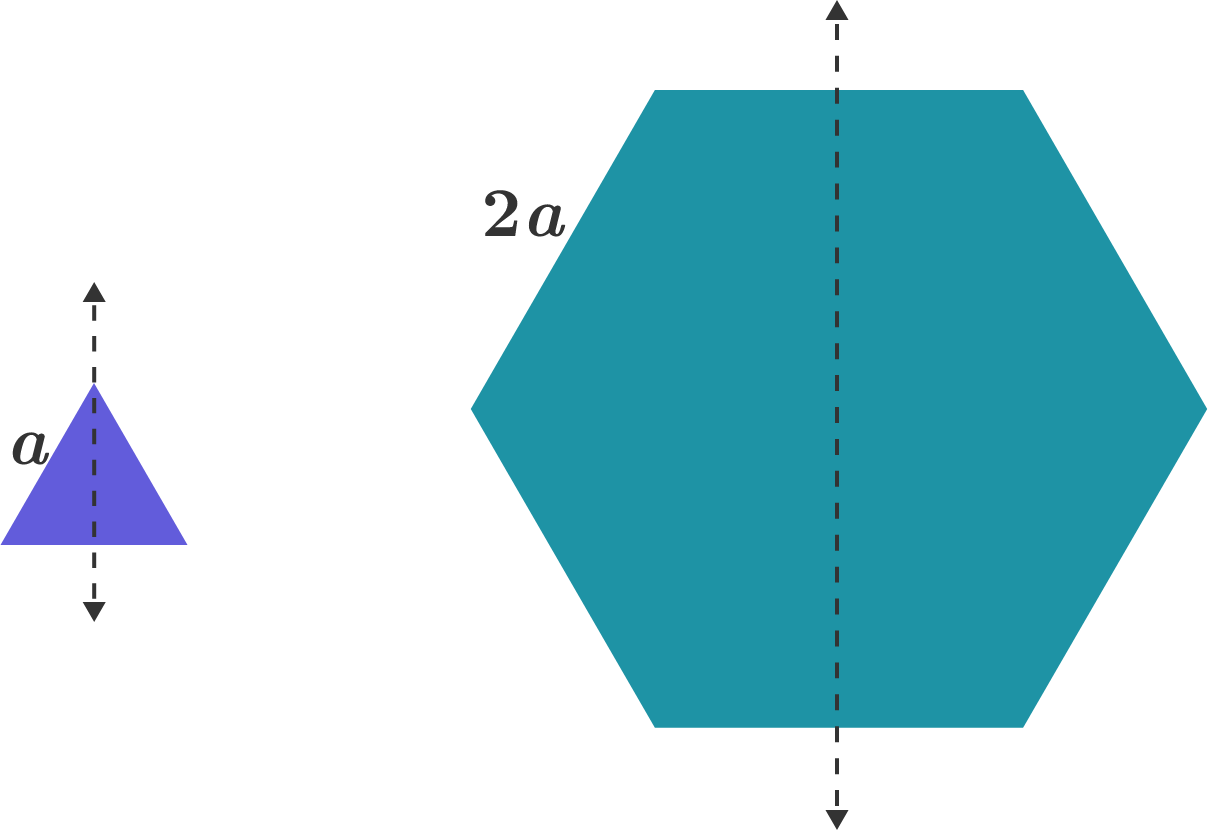# Co-relating moments of inertia with geometry.

An equilateral triangular plate has side length $a$, and the moment of inertia about the axis shown is $I$.

A regular hexagonal plate has side length $2a$, and the moment of inertia about the axis shown can be given as a constant multiple of $I$: $k I$.

What is $k?$

Note: Assume both plates have negligible thickness.×

Problem Loading...

Note Loading...

Set Loading...